# VOLUME OF RIGHT CIRCULAR CONE

##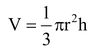Where V =  volume of cone

r =  radius of base of cone

h =  height of cone

question. Find the curved surface area of a right circular cone whose slant height is 10 cm and base radius is 7 cm.

Solution: Curved surface area = πr

= 22/7× 7 × 10 cm2

= 220 cm2

question. The height of a cone is 16 cm and its base radius is 12 cm. Find the curved surface area and the total surface area of the cone (π = 3.14).

Solution: Here, h = 16 cm and r = 12 cm

So, from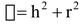we have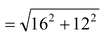cm = 20 cm

Curved surface area = πrh

= 3.14 × 12 × 20 cm2

= 753.6 cm2

Total surface area = πrh + π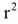=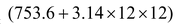cm2

= (753.6 + 452.16) cm2

= 1205.76 cm2

question. The height and the slant height of a cone are 21 cm and 28cm respectively. Find the volume of the cone.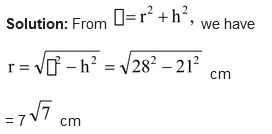So, volume of the cone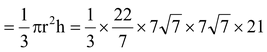cm3

= 7546 cm3

question. The diameter of a cone is 14 cm and its slant height is 9 cm. Find the area of its curved surface.

Solution: Curved surface of a right circular cone of radius r and slant height  h  is given by

S = πrh

Here,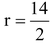cm = 7 cm and  h= 9 cm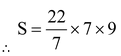cm2 = 198 cm2